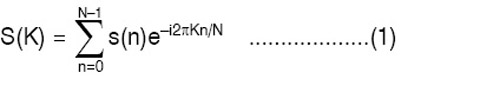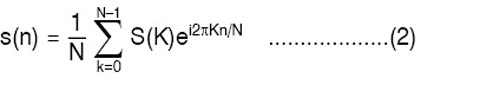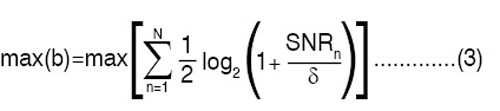Another variant of ADSL that does not necessitate the need of a POTS splitter is known as splitter-less ADSL and is defined in G.992.2 or G.Lite. ADSL Lite provides downstream speeds of 1.5Mbps and upstream speeds of 512kbps. Reduced data rates cause less interference in POTS signal, which provides freedom from a splitter at subscriber’s premises. So, it is possible to access the Internet by connecting G.Lite modems directly to phone jacks. A typical ADSL Lite set-up is shown in Fig. 2. This lower-version of ADSL results in significant cost-saving that can be passed on to subscribers. The plug-n-play functionality makes ADSL Lite more attractive to subscribers.

ADSL communication is a full-duplex communication system that is achieved on a pair of wires either by frequency division duplex (FDD), time division duplex (TDD) or echo-cancelling duplex (ECD). In FDD, two separate frequency bands are used, namely, upstream and downstream frequency bands. The modulation technique used in ADSL is either orthogonal frequency division multiplexing (OFDM) or discrete multi-tone (DMT). OFDM and DMT are almost the same thing. The similarities between OFDM and DMT are:
1. OFDM and DMT are both multicarrier communication, implemented digitally by means of a fast Fourier transform (FFT).
2. All sub-channels are orthogonal in both cases.
3. In both cases, the use of short cyclic prefix on the transmit signal allows perfect reconstruction of data at the transmitter.

The main differences between OFDM and DMT are:
1. DMT refers to baseband wire-line multicarrier communication, while OFDM is wireless bandpass multicarrier communication.
2. Because of the slowly varying nature of the channel, DMT allows spectral shaping by bit-loading to take advantage of measured channel characteristics.

Scientists at Stanford University and Bellcore have chosen DMT for ADSL, while OFDM has been adopted for wireless communication. There is no reason why DMT and OFDM could not be interchanged in their usage.

Actually, DMT implemented by FFT is orthogonal FDM. On the other hand, OFDM implemented by FFT is discrete multi-tone. In fact, there is no reason why OFDM should not refer to discrete wavelet multi-tone, wavelet packet modulation and any modulation based on orthogonal-filter banks since these all implement OFDM.

It is well-known from Fourier theory that, any kind of signal can be broken-up into its individual frequency components. By utilising this mathematical concept, spectral components of any signal can be obtained from a filter. The centre frequency of the filter will decide the output signal from the filter. Thus, it is possible to obtain all or some frequency components of a signal by utilising several filters of required centre frequency.

The algorithm used to transform digital signals (in time-domain) into corresponding spectral components (in frequency-domain) is known as fast Fourier transform. Mathematically, FFT of a digital signal s(n) is given by:where, K=0, 1,…………..,(N-1)

Inverse fast Fourier transform (IFFT) is used to convert frequency-domain signal into its corresponding time-domain digital signal and is given by:where, n=0, 1,……………,(N-1)

Several modulation techniques are available for DSL technologies like carrierless amplitude modulation (CAP), multiple virtual line (MVL), OFDM and DMT. In ADSL, however, DMT is most widely used to utilise the 6MHz bandwidth available on the unshielded copper pair to provide high-speed Internet access. The usable frequency range is separated into 256 frequency bands or carrier channels, each of 4.3125kHz.

Each carrier is then quadrature amplitude modulated to carry two bits per second. By varying the number of bits per symbol within a channel, the modem can be rate-adaptive. This is an important feature of DMT that provides a possibility to allocate the number of bits per carrier according to its corresponding signal-to-noise ratio (SNR). This is known as bit-loading and is of two types: margin-adaptive and rate-adaptive. Margin-adaptive algorithm minimises the bit error rate (BER) for a given bit-rate.

On the other hand, rate-adaptive algorithm maximises the bit-rate for a fixed BER and pre-defined power constraints. The rate-adaptive bit-loading is a reformulation of Shannon capacity formula, which gives the total achievable bit-rate b in N number of sub-channels as:But,

SNRn = Enhn ………………(4)

where, hn represents subcarrier SNR when unit energy is applied, En is the allocated energy per subcarrier, and is the difference between SNR needed to achieve maximum Shannon capacity and SNR to achieve this capacity at a given bit-error probability.